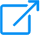teamviewer.comor

# Is there a way to generate TeamViewer Passwords in Excel?

I am attempting to make Excel generate a 6-character password string, exactly like TeamViewer (3 letters, 3 numbers). Is there a function I might be unaware of?

I have tried `=CHOOSE(RANDBETWEEN(1,2),CHAR(RANDBETWEEN(0,9)),CHAR(RANDBETWEEN(97,122)))&CHOOSE(RANDBETWEEN(1,2),CHAR(RANDBETWEEN(0,9)),CHAR(RANDBETWEEN(97,122)))&CHOOSE(RANDBETWEEN(1,2),CHAR(RANDBETWEEN(0,9)),CHAR(RANDBETWEEN(97,122)))&CHOOSE(RANDBETWEEN(1,2),CHAR(RANDBETWEEN(0,9)),CHAR(RANDBETWEEN(97,122)))&CHOOSE(RANDBETWEEN(1,2),CHAR(RANDBETWEEN(0,9)),CHAR(RANDBETWEEN(97,122)))&CHOOSE(RANDBETWEEN(1,2),CHAR(RANDBETWEEN(0,9)),CHAR(RANDBETWEEN(97,122)))`, and here's an example of one of the results: `ckjfs`.

Please see above for the Formula.

The expected result is something like: `aaa111`, or `1aaa11`. I don't want the Formula to allow something like `11aaaa``aaaaaa`, or `1234aa`.

Tagged:

• @AhmedAlik Here is an option for you to consider:

https://imgur.com/S97xQ2w

Formula in `A2`:

```=RANDBETWEEN(1,6)
```

Formula in `B2`:

```=CHAR(RANDBETWEEN(IF(OR(RANK.EQ(A2,\$A\$2:\$A\$7)+COUNTIF(\$A\$2:A2,A2)-1={1,2,3}),48,97),IF(OR(RANK.EQ(A2,\$A\$2:\$A\$7)+COUNTIF(\$A\$2:A2,A2)-1={1,2,3}),57,122)))
```

Drag down.....

Formula in `D2`:

Excel 2016 with `CONCAT`:

```=CONCAT(C2:C7)
```

Lower versions without `CONCAT`:

```=C2&C3&C4&C5&C6&C7
```

• @AhmedAlik I can Offer this rather long array formula:

```=ArrayFormula(TEXTJOIN("",TRUE,IF(MID(TEXT(DEC2BIN(INDEX({7,11,13,14,19,21,22,25,26,28,35,37,38,41,42,44,49,50,52,56},RANDBETWEEN(1,20))),"000000"),{1,2,3,4,5,6},1)="0",
CHAR(CHOOSE({1,2,3,4,5,6},RANDBETWEEN(48,57),RANDBETWEEN(48,57),RANDBETWEEN(48,57),RANDBETWEEN(48,57),RANDBETWEEN(48,57),RANDBETWEEN(48,57))),
CHAR(CHOOSE({1,2,3,4,5,6},RANDBETWEEN(97,122),RANDBETWEEN(97,122),RANDBETWEEN(97,122),RANDBETWEEN(97,122),RANDBETWEEN(97,122),RANDBETWEEN(97,122))))))
```

I had to test it in Google Sheets because I only have an old version of Excel without the array concatenation features - it should work in later versions of Excel if you remove the ArrayFormula wrapper and enter it with Ctrl-Shift-Enter.

The idea is that there are only 20 ways of selecting 3 items (letters) out of 6 (letters and numbers) so choose one of them in binary (e.g. 010101) and generate letters where there are 1's and numbers where there are 0's.